# Volume + system of equations - math problems

#### Number of problems found: 46

• Consecutive membersThe block has a volume of 1728 cm³. Determine the lengths of the edges a, b, c of the blocks for which a
• Barrel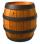The barrel is filled with water up to 57% of its capacity. When we add two buckets will be filled to 73% of its capacity. What is the volume of a barrel, if the volume of the bucket is 12 liters?
• Cuboid - complicatefThree walls of the same cuboid has content 6 cm2, 10 cm2 and 15 cm2. Calculate the volume of the cuboid.
• Block or cuboidThe wall diagonals of the block have sizes of √29cm, √34cm, √13cm. Calculate the surface and volume of the block.
• Reservoir + waterReservoir completely filled with water weighs 12 kg. After pouring off three quarters of the amount of water weights 3 kg. Calculate the weight and volume of the reservoir.
• CuboidCuboid with edge a=6 cm and space diagonal u=31 cm has volume V=900 cm3. Calculate the length of the other edges.
• Tank 9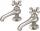The tank with volume V is filled with one pump for three hours and by second pump for 5 hours. When both pumps will run simultaneously calculate: a) how much of the total volume of the tank is filled in one hour b) for how long is the tank full
• Solid cuboidA solid cuboid has a volume of 40 cm3. The cuboid has a total surface area of 100 cm squared. One edge of the cuboid has a length of 2 cm. Find the length of a diagonal of the cuboid. Give your answer correct to 3 sig. Fig.
• Water pool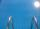Pool with volume 990hl completely filled, if water flows by one tap 8 hours and by second tap 6 hours. First tap give 10hl more than second per hour. How many hl flows in each of them in an hour?
• A bottleA bottle full of cola weighs 1,320 g. If we drink three-tenths of it, it will weigh 1,008g. How much does an empty bottle weigh?
• RingThe ring made from gold and copper alloy has a weight of 14.5 g and a volume of 1.03 cm3. How much gold and how much copper it contains? The metal densities are Au 19.3 g/cm³ and Cu 8.94 kg·dm-3
• Bottles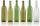Mrs. Violet brewed 15 l of raspberry juice. She poured it into 17 bottles. Some were 3/4 liter and some one liter. How many was bottles of this two types?
• BucketThe bucket half filled with water weighs 5.55 kg, the full bucket weighs 9.85 kg. How much does the bucket weigh?
• Two pipes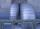One pipe fill one-fifth volume 20 minutes before by second one. The two pipes together will fill the tank in two hours. How long is will fill tank each pipe separately?
• BottlesThe must is sold in 5-liter and 2-liter bottles. Mr Kucera bought a total of 216 liters in 60 bottles. How many liters did Mr. Kucera buy in five-liter bottles?
• Body diagonalThe cuboid has a volume of 32 cm3. Its side surface area is double as one of the square bases. What is the length of the body diagonal?
• Barrel with water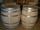The water barrel has a weight of 52 kg. When we pour a quarter of the water, the weight of the barrel is reduced to 40 kg. Determine the weight of the empty barrel.
• Gasoline tank 2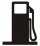A gasoline tank is 1/6 full. When 25 liters of gasoline were added, it became 3/4 full. How many liters are more needed to fill them? Show your solution.
• SolutionsHow much 60% solution and how much 35% solution is needed to create 100 l of 40% solution?
• In the dairy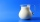There were three times more packages of milk in the dairy than half a liter. When sold 10 liter and ten half-liter containers, four times more liters remained than half-liter packages. How many packages were there originally?

Do you have an interesting mathematical word problem that you can't solve it? Submit a math problem, and we can try to solve it.

We will send a solution to your e-mail address. Solved examples are also published here. Please enter the e-mail correctly and check whether you don't have a full mailbox.

Please do not submit problems from current active competitions such as Mathematical Olympiad, correspondence seminars etc...

Do you have a system of equations and looking for calculator system of linear equations? Tip: Our volume units converter will help you with the conversion of volume units. See also more information on Wikipedia.